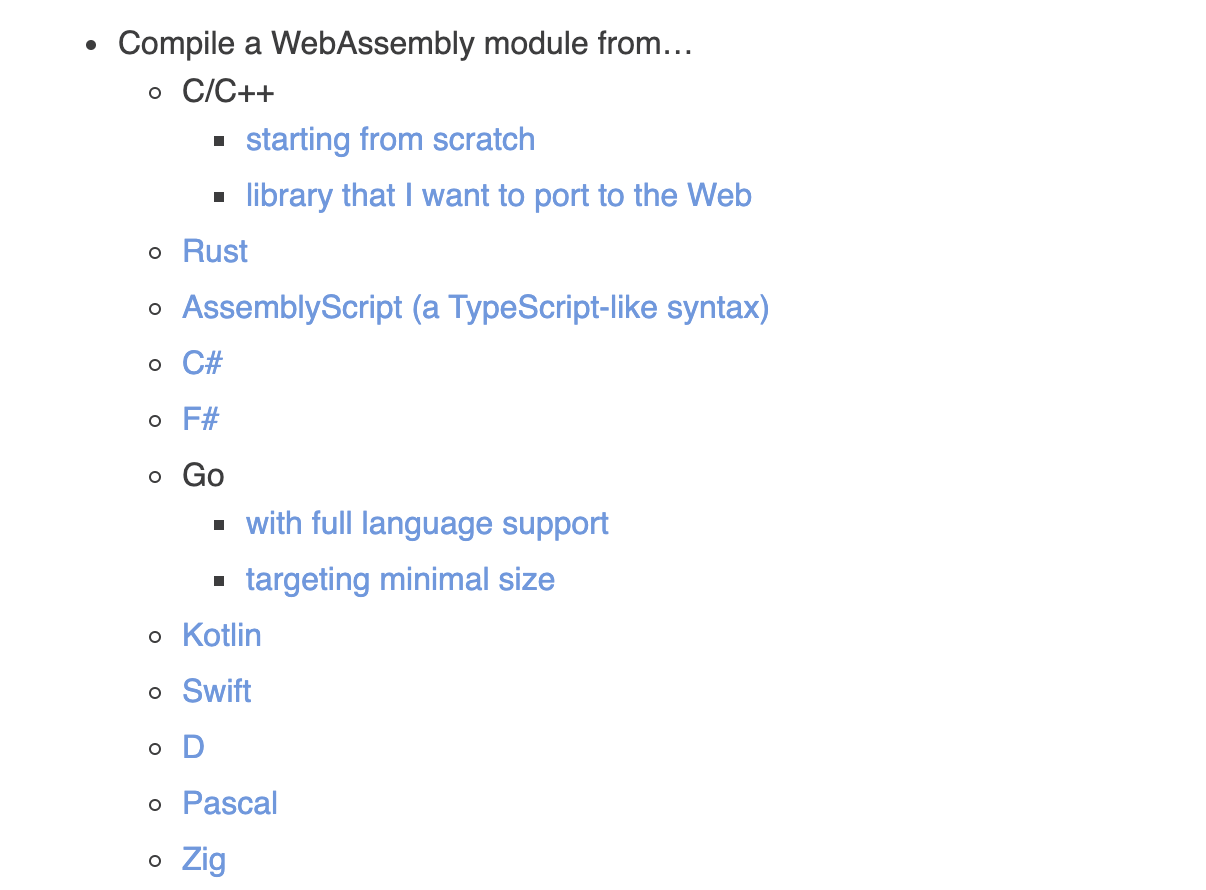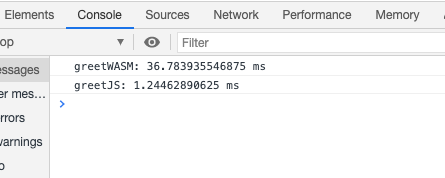# WebAssembly With Rust

4 大主流浏览器已经都支持了 WebAssembly，同时也很多项目中得到了实际应用，本文将分享如何构建一个 WebAssembly (简称 WASM) 以及在浏览器中的使用。

## 使用 Rust 构建一个 WASM### 基础环境初始化

``````curl --proto '=https' --tlsv1.2 -sSf https://sh.rustup.rs | sh
``````
1. 初始化一个 WASM 项目
``````cargo new --lib hello-wasm
``````

### 如何将 Rust 构建为 WASM

Rust 社区中提供了封装好的工具wasm-pack，可以直接将 rust 的代码打包为一个 npm 包

``````wasm-pack build -t web
``````

``````<script type="module">
import init, { greet } from './pkg/hello_wasm.js'
init().then(() => {
greet('WASM')
})
</script>
``````

## WASM 是如何和 Web 进行交互的

### 加载 WASM

`wasm-pack` 已经直接帮我们打包好了对应的模块，可以直接在浏览器中是用原生 import

``````<script type="module">
import init, { greet } from './pkg/hello_wasm.js'
init().then(() => {
greet('WASM')
})
</script>
``````

#### pkg/hello_wasm.js

``````fetch('hello_wasm_bg.wasm').then(async (module) => {
let imports = {}
let wasm = await WebAssembly.instantiate(module, imports)

// wasm 这个就是 WebAssembly 的实例了
return wasm
})
``````

#### 调用 WASM 中的方法

``````<script type="module">
import init, { greet } from './pkg/hello_wasm.js'
init().then(() => {
greet('WASM')
})
</script>
``````

``````export function greet(name) {
var ptr0 = passStringToWasm0(
name,
wasm.__wbindgen_malloc,
wasm.__wbindgen_realloc
)
var len0 = WASM_VECTOR_LEN
wasm.greet(ptr0, len0)
}
``````

#### WASM 中如何调用 Web 中的方法

Rust 中的代码，借助`wasm_bindgen`通过 `extern "C"` 定义了要从外部引入的函数

``````#[wasm_bindgen]
extern "C" {
}

#[wasm_bindgen]
pub fn greet(name: &str) {
}
``````

``````fetch('hello_wasm_bg.wasm').then(async (module) => {
let imports = {}
imports.alert = function (arg0, arg1) {
// 获取到内存区域
}
// 通过 imports 注入
let wasm = await WebAssembly.instantiate(module, imports)

// wasm 这个就是 WebAssembly 的实例了
return wasm
})
``````

## WASM 通信的性能消耗

``````
#[wasm_bindgen]
pub fn greet(name: &str) -> String {
let mut result: String = "prefix_".to_owned();
result.push_str(name);

return result;
}
``````

``````<script type="module">
import init, { greet } from './pkg/hello_wasm.js'
init().then((wasm) => {
console.time('greetWASM')
for (let i = 0; i < 20000; ++i) {
greet('Test')
}
console.timeEnd('greetWASM')

function greetJs(str) {
return 'prefix_' + str
}
console.time('greetJS')
for (let i = 0; i < 20000; ++i) {
greetJs('Test')
}
console.timeEnd('greetJS')
})
</script>
``````#tech